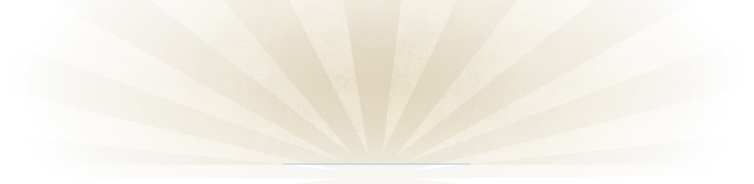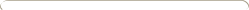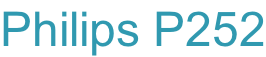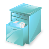﻿ Philips P252Details:

The P252 was a very capable printing calculator. Besides the four standard operations it calculated the square as well as the square-root of a number.  Numbers and status information are printed with a dot-matrix printer (one of the very first?).  Furthermore it contained two independent core memories to store and retrieve numbers at any moment during a calculation. The type of memory retains its content also after switching the power off and on.

It was capable but also large and heavy;  larger for example than the Friden 1217, almost as heavy and ........  1.5  times the price of the Friden.   However, compared to that mechanical machine it is very quiet, very easy to operate, more versatile and results are printed almost instantly.

Usage:

Enter the 1st number, then pushing  “ + “ adds the number to the result register.

= “ prints a total.  It does not clear the result register.

- “ subtracts similarly.

Multiplication:

Enter the 1st number, push the “X” key, enter the 2nd number, push the “=” key.

Division:

Similar to multiplication using the “:” key.

Function of some keys, switches etc.

• " i " / "ii" selects one of two memory locations
• " ^ "  adds last printed number to  memory
• " ^ “ subtracts the last printed number from memory
• " v "  retreives the current value from memory and clears the memory
• " "  is a memory recall (leaves the memory contents unchanged)
• "C" corrects any input that hasn’t been printed yet
• To multiply with a constant K:
• - Put constant K in memory i or ii
• - Push the  ‘X’  key
• - Enter 1ste number,  then push ‘=’ (result is printed)
• - Enter 2nd number,  then push ‘=’   (result is printed)
• - Etc.
• " "  calculates the square root of the last printed number
• % ”, followed by “X” cause the divisor divided by 100 to operate on the last printed value
• The number wheel sets the number of digits behind the comma between 0 and 6Click to view a full size picture (opens in a new tab)Serial number:

Built in:

Functions:

Printing speed

Market-introduction:

Production until:

Price:

5001

1970